Courses

# End Point And Equivalence Point - Mole Concept Chemistry Notes | EduRev

## Chemistry : End Point And Equivalence Point - Mole Concept Chemistry Notes | EduRev

The document End Point And Equivalence Point - Mole Concept Chemistry Notes | EduRev is a part of the Chemistry Course Physical Chemistry.
All you need of Chemistry at this link: Chemistry

Titration is used in analytical chemistry to determine acid, bases, reductants, oxidants, and other species. Titrations can usually occur in reactions such as redox reactions and acid-base reactions. During the process, two important stages known as endpoint and equivalence point are reached:

End Point

The point in titration, usually indicated by a change of color of an indicator, at which a particular reaction is complete, is called end point. It is an experimental value.

Equivalence Point

The equivalence point represents the state when the equivalent of a reacting species becomes equal to the equivalent of other reacting species. It is a theoretical value.
End point is almost near to equivalence point.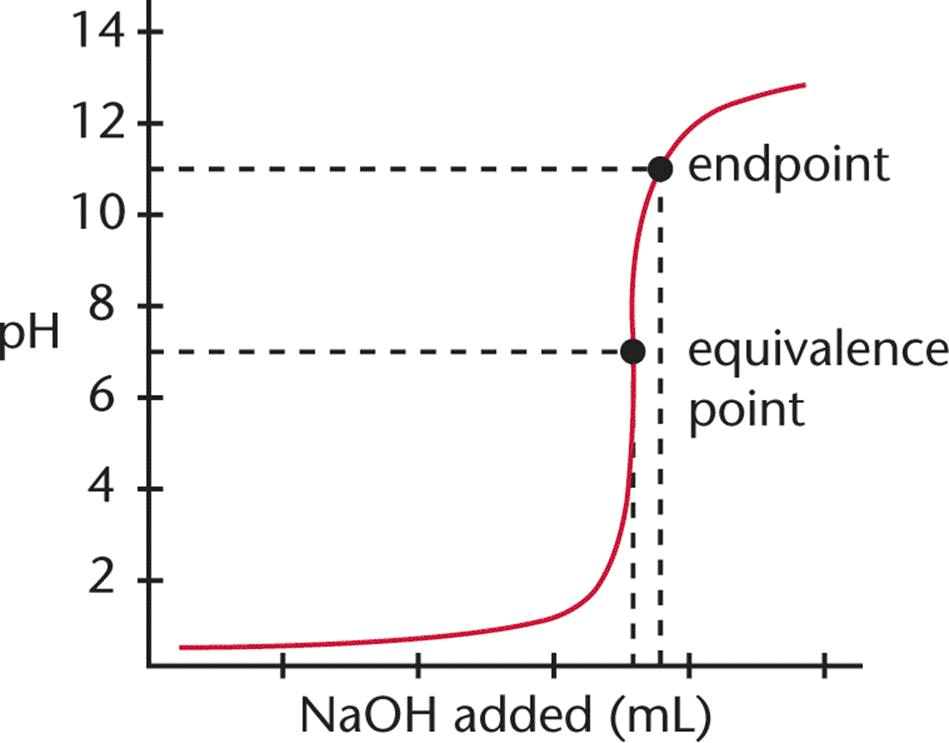Difference Between Endpoint and Equivalence Point

• Although the endpoint is normally regarded as the equivalence point, they are not the same.
• But since there is only a slight difference between an equivalent point and an endpoint, it can be considered the same for laboratory purposes.
• The main difference between an equivalence point and an endpoint is that the former marks the end of the reaction whereas the latter is a point where the indicator changes colour.
 Endpoint Equivalence Point Point where the indicator changes colour The point at which the titrant is chemically equivalent to the analyte in the sample Comes after the equivalence point Comes before the endpoint Weak acids can have only one endpoint Weak acids can have multiple equivalence point

Indicators

There are the substances, the by a sharp colour change, indicate the completion of a chemical reaction.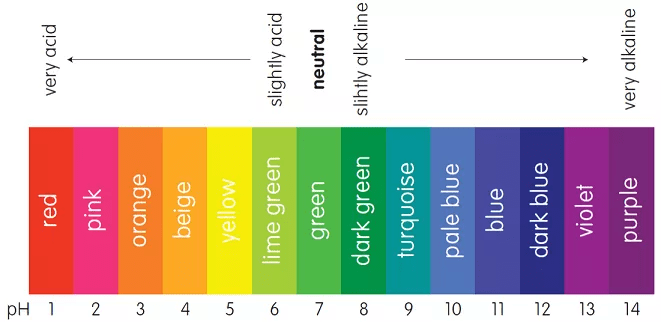pH scale used as an indicator

• Indicators for titration of acids and bases are usually weak organic acids or bases, yielding ions of a different color from the unionized molecule, e.g., litmus is red with acids and blue with alkalies, a change in color indicates that neutralization is complete.
• On the basis of different titrations, indicators are classified into several types, viz.,
(a) neutralization indicators (acid-base or pH indicators,
(b) oxidation-reduction indicators,
(d) mixed indicators,
(e) screened indicators,
(f) metal indicators and
(g) universal indicators

Double Indicators Titrations for Two End Points

A double indicator titration is a process used in chemistry experiments to determine and analyze the amount and concentration of acids or bases in a solution at two endpoints.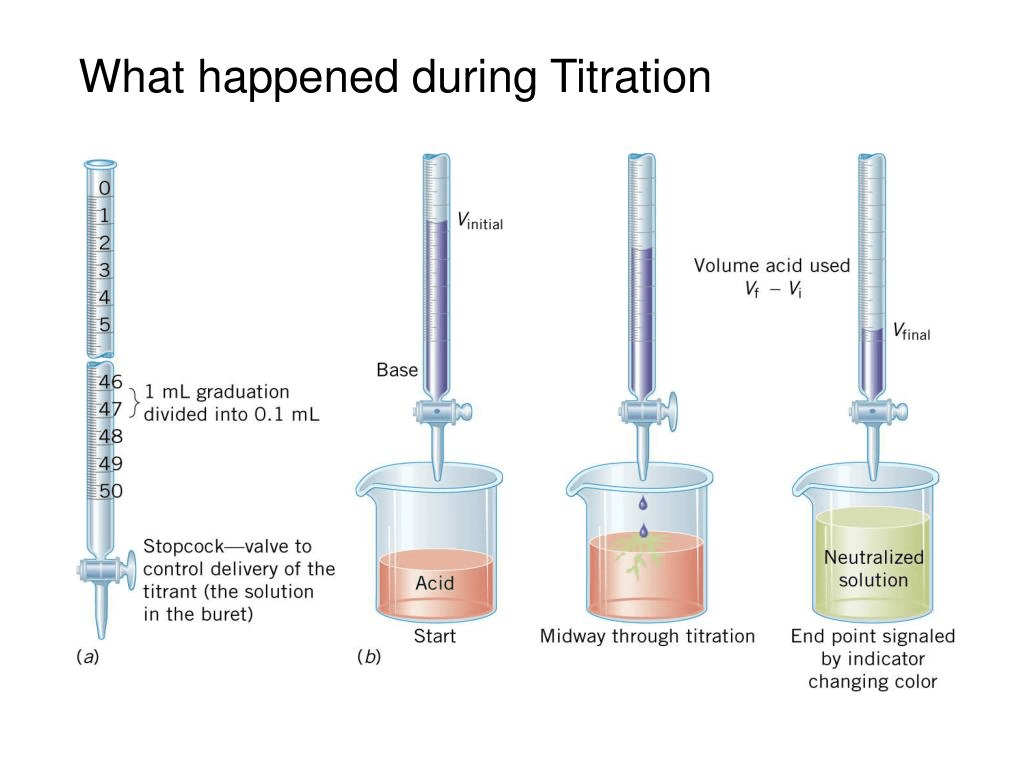• For the titration of alkali mixtures (e.g., NaOH + Na2CO3) or (Na2CO3 + NaHCO3), two indicators phenolphthalein and methyl orange are used.
• Phenolphthalein is a weak organic acid and gives an endpoint between pH 8 to 10, while methyl orange, a weak base itself indicates an endpoint sharply between pH 3.1 to 4.4.

The following points must, therefore, be remembered.

•  Phenolphthalein is not a good indicator for weak alkali titrations.
•  Methyl orange is not a good indicator for weak acid titrations.
• In the case of strong acid vs. Strong base titrations using phenolphthalein or methyl orange as an indicator, the equivalent point suggests that meq. Of acid = meq. Of alkali.
• In the case of strong acid vs. Strong base such as Na2CO3 (which acts as a strong base in I step of dissociation and weak base in II step dissociation), using phenolphthalein as indicator, the meq. Of acid are titrated only up to NaHCO3 state.
Na2CO3 +  H+ → NaHCO3 + Na+
i.e., meq. Of acid = 1/2 meq. Of Na2CO3
(if Eq. Mass of Na2CO3 = M/2)
Or
meq. Of acid = meq. Of Na2CO3
(if Eq. Mass of Na2CO= M/1)
However, titration with methyl orange as indicator, the meq. Of acid corresponds of total meq. Of alkali present at that time in mixture.
• One should be careful in calculating meq. Of alkalies, if methyl orange is used as an indicator in titration, i.e., for fresh mixture or in continuation of phenolphthalein, e.g., Na2CO3 + NaOH mixture v.s HCl

Case I: I endpoint determined using phenolphthalein as an indicator and then methyl orange is used to get II endpoint in continuation.
Case II: Endpoint is determined using phenolphthalein as an indicator. Next time endpoint is determined by taking another (fresh) same volume of the mixture using methyl orange as an indicator.

For Phenolphthalein:
Meq. Of Acid = meq. Of NaOH = 1/2 meq. Of Na2CO3

For Methyl Orange: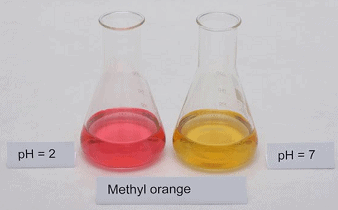Different colors of methyl orange at different pHMeq. Of acid = meq. Of NaOH = meq. Of Na2CO3

Example: 200 mL of a solution of mixture NaOH and Na2CO3 was first titrated with phenolphthalein and N/10 HCl. 17.5 mL of HCl was required for the endpoint. After this methyl orange was added and 2.5 mL of the same HCl was again required for the next endpoint. Find out the mass of NaOH and Na2CO3 in the mixture:

Solution:
Phenolphthalein as an indicator:
Meq. Of HCl used for 200 mL solution = 17.5 x (1/10) = 1.75
∴ meq. Of NaOH + 1/2 meq. Of Na2CO3 = 1.75
Methyl Orange as indicator: After I end point, methyl orange is added
1/2 meq. Of Na2CO= meq. Of HCl used

= 2.5 x (1/10) = 0.25

By Eqs.
Meq. Of NaOH = 1.75 – 0.25 = 1.50
∴ (w/40) x 1000 = 1.50
Or WNaOH = 0.06 g per 200 mL
Also, meq. Of Na2CO3 = 0.25 x 2 = 0.50
∴ (w/53) x 1000 = 0.50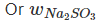= 0.0265 g per 200mL

Eudiometry

The technique involving volume measurement during the reaction of gases is called eudiometry.

• Since volume of gas, V ∝ number of mole at constant P, T and thus, volume ratio of gases can be directly used in place of mole ratio to analyse the given data.

Example: A mixture of ethane (C2H6) and ethene (C2H4) occupies 40 litres at 1.00 atm and at 400 K. The mixture reacts completely with 130 g of Oto produce CO2 and H2O. Assuming ideal gas behavior, calculate the mole fractions of C2H4and C2H6 in the mixture.

Solution: For a gaseous mixture of C2H6 and C2H4
PV = nRT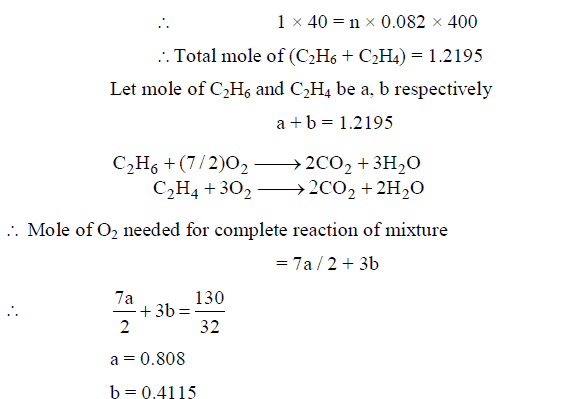∴ Mole fraction of C2H6 = 0.808 / 1.2195 = 0.66

And Mole fraction of C2H= 0.34

To Represent the Concentration of H2O2 Solution:

In percentage: The mass of H2O2 present in 100 mL H2Osolution is H2O2 concentration in the percentage of H2O2 solution.

In volume: The volume of Oat STP given by 1 mL H2Osolution on decomposition is H2Oconcentration of H2O in volume.
Direct conversions can be made by using the following relations

• % strength 17/56 x  volume strength
• Volume strength = 5.6 x Normality
• Volume strength = 11.2 x Molarity

To Represent the Concentration of Oleum:

(100 + X%) of oleum means ‘X’ g H2O reacts with equivalent amount of free SO3 to give H2SO4.

Example: Calculate the % of free SO3 in oleum (a solution of SO3 in H2SO4) that is labeled 109% H2SO4)

Solution: percentage above 100 represents the mass of H2O that reacts with dissolved SO3 in oleum to give H2SO4, i.e., 9 g H2O reacts with free SOto produce H2SO4

18 g H2O reacts with 80 g SO3

∴9 g H2O will react with 40 g SO3
Or % of free SO3 = 40

To Determine Hardness of Water:

• Water, which gives foams easily with soap is called soft water and if not then hard water.
• The hardness of water is due to the presence of bicarbonates, chlorides, and sulphates of Ca and Mg.
• The hardness is temporary due to bicarbonates and permanent due to chlorides and sulphates of Ca and Mg
• The extent of hardness is known as degree of hardness defined usually as the number of parts by mass of CaCO3  present per million parts by mass of water:
• Hardness is expressed in ppm i.e., 1 ppm = 1 part of CaCOin 106 part of hard water.

The reason for choosing CaCO3 as the standard to express hardness, inspite of the fact that CaCO3 is not soluble in water but its molar mass is 100 which makes calculation easy.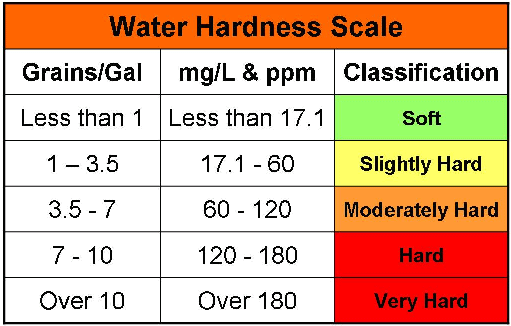Water hardness scale

Water Softeners

The hardness may be removed by either of the reactants on treating with water.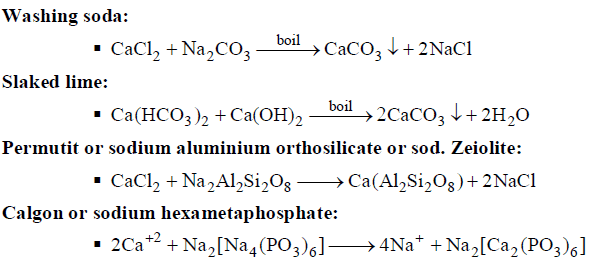Offer running on EduRev: Apply code STAYHOME200 to get INR 200 off on our premium plan EduRev Infinity!

## Physical Chemistry

84 videos|106 docs|31 tests

,

,

,

,

,

,

,

,

,

,

,

,

,

,

,

,

,

,

,

,

,

;